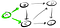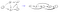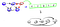# Algorithms on Graphs: Simplifying DAGs with Topological Sort

One of the difficulties in dealing with directed acyclic graphs (DAGs) is that they can look complicated and messy. This makes it difficult to see the relationships that vertices have with each other.

In this post, we’re going to discuss and learn how to rearrange the vertices of DAGs in such a way that will allow us to simplify these graphs and see possible patterns much easier.

# Source and Sink Vertices

Before we start, we need to be familiar with some terms: source and sink vertices.

A source vertex is simply a vertex where no edges point towards it. For example:We’ll use green to highlight a source vertex

Vertex A has edges that only flow out, so by definition it is a source vertex. Now, there are graphs that can have multiple source vertices such as this one:

While source vertices have edges that only point outwards, sink vertices are the exact opposite. They’re vertices with edges that only flow into them.

In this example, vertex E doesn’t have any edges putting outwards but have two pointing into it. This illustrates that if one starts a traversal at a sink, it would not be able to get out.

Just like source vertices, there can be multiple sinks in a graph as well:

One way to think about sinks is that they’re analogous to actual sinks, where water flows in but is unable to flow back out.

# Topological Sort

It’s important to note that topological sort (aka top sort) only works on DAGs and while it isn’t really categorized as a sorting algorithm like Merge Sort and Quick Sort, it does have some similarities with them.

Top sort “sorts” vertices in such a way that edges from the vertices flow in one direction. Let me illustrate what I mean by this. Here’s an example:From original DAG to Top Sorted DAG

Notice that the order of the vertices are sorted in such a way that the edges all point in one direction (to the right). The source vertex is on the left while the sink is to the right.

Top sort can be thought of as a way to simplify how we view the overall graph. The result is still a DAG but it looks much simpler because we can clearly see the flow of the edges and how the edges connect to the vertices. Let’s look at a DAG that‘s a bit more complicated:

From a first glance, the first DAG is compact and have edges going left, right, and down. But once sorted, the DAG looks much cleaner and we can see the paths from each vertex.

What I really want to stress is that top sort simplifies our DAGs in such a way that we can better see the flow of the edges. This makes it easier to understand which vertices can reach which vertices.

What distinguishes top sort from the other sorting algorithms is that the ordering of the elements (vertices) can be different but only in such a way that it remains consistent to the flow of the edges. Let’s look this example:

Notice how switching the vertices A and C doesn’t alter the flow of the edges in any way. The flows still goes in one direction (source to sink) and both results are still DAGs. Therefore, they’re both valid results of top sort.

Our approach to top sort will utilize our understanding of source and sink vertices. The idea is that we’ll use Depth-First Search (DFS) to help us find our sinks. Upon finding our sinks, we’ll add them to the end of an array and continue this process until we fill up the entire array.

Let’s look at an example. Suppose we have this graph from earlier:

We could technically start at any vertex but let’s start at vertex A to illustrate our DFS method. Starting from A, we do a DFS on its neighbors B and D. Let’s start with B:

As we do a DFS on B, we run into a sink vertex, D. Since D is a sink, we add it to the end of our result array and we continue with the other neighbors of B. Since E only has one neighbor and D has already been processed, E is added to the array.

We continue on with this process finishing our DFS on A:

Since all our vertices have been processed when we did DFS on A, we are left with C as the only non-visited vertex. So we apply DFS on C and get the result:Since the neighbors of C have already been visited, then C is added to the next open spot in the array

Here’s the code in JavaScript:

Since we’re just doing DFS on all the unvisited vertices, the runtime is O(V + E) and the space complexity is O(V).

# Summary

• Source vertices are any vertices with only outward edges. There can be many source vertices in a graph.
• Sink vertices are vertices that only have inward edges. There can also be many sink vertices in a graph.
• Topological sort (top sort) sorts vertices in an ordering such that the edges from the vertices flow in one direction.
• Top sort simplifies the DAGs to show clearer relationships between vertices.
• Top sort has a runtime of O(V +E ) and a space complexity of O(V).

## More from Try Khov

Software Engineer